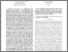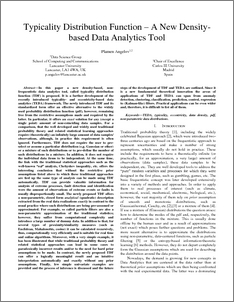# Typicality distribution function:a new density-based data analytics tool

Angelov, Plamen (2015) Typicality distribution function:a new density-based data analytics tool. In: Neural Networks (IJCNN), 2015 International Joint Conference on. IEEE, IRL, pp. 1-8.Preview
PDF (PID3688517)
PID3688517.pdf - Accepted Version

## Abstract

In this paper a new density-based, non-frequentistic data analytics tool, called typicality distribution function (TDF) is proposed. It is a further development of the recently introduced typicality- and eccentricity-based data analytics (TEDA) framework. The newly introduced TDF and its standardized form offer an effective alternative to the widely used probability distribution function (pdf), however, remaining free from the restrictive assumptions made and required by the latter. In particular, it offers an exact solution for any (except a single point) amount of non-coinciding data samples. For a comparison, that the well developed and widely used traditional probability theory and related statistical learning approaches require (theoretically) an infinitely large amount of data samples/ observations, although, in practice this requirement is often ignored. Furthermore, TDF does not require the user to pre-select or assume a particular distribution (e.g. Gaussian or other) or a mixture of such distributions or to pre-define the number of such distributions in a mixture. In addition, it does not require the individual data items to be independent. At the same time, the link with the traditional statistical approaches such as the well-known “nσ” analysis, Chebyshev inequality, etc. offers the interesting conclusion that without the restrictive prior assumptions listed above to which these traditional approaches are tied up the same type of analysis can be made using TDF automatically. TDF can provide valuable information for analysis of extreme processes, fault detection and identification were the amount of observations of extreme events or faults is usually disproportionally small. The newly proposed TDF offers a non-parametric, closed form analytical (quadratic) description extracted from the real data realizations exactly in contrast to the usual practice where such distributions are being pre-assumed or approximated. For example, so call- d particle filters are also a non-parametric approximation of the traditional statistics; however, they suffer from computational complexity and introduce a large number of dummy data. In addition to that, for several types of proximity/similarity measures (such as Euclidean, Mahalonobis, cosine) it can be calculated recursively, thus, computationally very efficiently and is suitable for real time and online algorithms. Moreover, with a very simple example, it has been illustrated that while traditional probability theory and related statistical approaches can lead in some cases to paradoxically incorrect results and/or to the need for hard prior assumptions to be made. In contrast, the newly proposed TDF can offer a logically meaningful result and an intuitive interpretation automatically and exactly without any prior assumptions. Finally, few simple univariate examples are provided and the process of inference is discussed and the future steps of the development of TDF and TEDA are outlined. Since it is a new fundamental theoretical innovation the areas of applications of TDF and TEDA can span from anomaly detection, clustering, classification, prediction, control, regression to (Kalman-like) filters. Practical applications can be even wider and, therefore, it is difficult to list all of them.

Item Type:
Contribution in Book/Report/Proceedings